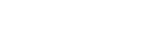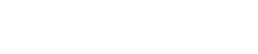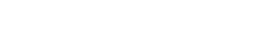# Population balance in Simcenter STAR-CCM+

What is a population balance and how is it used in Simcenter STAR-CCM+? This is something we will dig a bit deeper into in this week’s blog post. In Simcenter STAR-CCM+ there are mainly two population balance models, or size distribution models, that are used within the Eulerian framework: S-Gamma and AMUSIG, both of which can be used in the pure EMP simulations. S-Gamma can, as of the latest release (2021.2) also be used with MMP (mixture multiphase). The aim of this article is not to understand the differences between the different multiphase models in Simcenter STAR-CCM+, but to focus on the population balance models. A summary and description of the available multiphase models in Simcenter STAR-CCM+ is given in this previous  blog post: Multiphase modeling capabilities in Simcenter STAR-CCM+ – VOLUPE Software.

## What does as population balance do?

A population balance is needed when you have a dispersed phase in a continuous phase and is not explicitly modelling the dispersed phase, as would typically be the case with a smaller number of particles in e.g., some fluidized bed applications. It is then necessary to combine the mass and momentum transport equations with a population balance equation (PBE). The main task of the of the PBE is to keep track of the particle number density, and to describe the shift in size from different physical phenomena. Meaning that it keeps up to speed with how many particles you have a in size-range, typically in the range: (dp represent the particle diameter.)When looking at the general form of the PBE shown below, the terms B and D represent birth and death of a particle size within the size-range. The birth and death of the particles can be cause by breakup, coalescence, nucleation, dissolution etc. More about some of these physical phenomena later.There are two main ways of solving the PBE in Simcenter STAR-CCM+: using the S-gamma or AMUSIG method. Instead of solving for number density, the method of moments solves for a number of moments (S-Gamma in STAR-CCM+). The other method is the method of classes. The dispersed phase is split into several size classes. For each class, mass and momentum balances are solved separately (AMUSIG in STAR-CCM+).

## When should I use a population balance?

A population balance in CFD is typically a collection of equations describing a dispersed phase in a continuous phase. These equations are solved alongside the Navier-Stokes equations and accounts for a number of physical processes relating to the change in size of the dispersed phase. It should be used when the dispersed phase size distribution change due to interaction between the dispersed phase and the continuous phase, but also when there are interactions within the dispersed phase. One good example is the simulation of droplets and bubbles, where the sheer number of dispersed bubbles or droplets renders explicit modelling of the dispersed phase or phases mute.

A population balance can also be used when investigating the particle-size interaction with the continuous flow.

## The death and birth of the dispersed phase size groups

In this section we will go over the common terms that are involved with the destruction or creation of the dispersed phase size groups. First, we have the two opposite terms of breakup and coalescence, where breakup is the destruction of e.g., a droplet into smaller droplets and coalescence is the merging of smaller droplets into bigger ones from collisions. For laminar flow, both coalescence and breakup are driven by the shear rate of the continuous phase. While for turbulent flows, coalescence and breakup are driven by turbulent scales, of the continuous phase.

Coalescence has separate submodels for collision rate and for collision efficiency, basically models for how often particles collide and how “good” those collisions are. Understanding how “good” a collision relates to velocity of the particles colliding, the angle at which they collide and if the collision is head-on or grazing. The breakup model has submodels for breakage rate and fragments particle size distribution. It is important to know that all models have an overall calibration constant that you can adjust to suit your requirements. The critical Weber number is an adjustable parameter that is relevant for the breakup rate models.

Dissolution is where a solute dissolves into a solvent, the solute can be either gaseous, liquid or solid and the solvent is often a liquid. Nucleation is the opposite where in many cases a solid form in a solvent, e.g., crystallisation. Note that it can also relate to the creation of bubbles in a boiling case, then gases form in a liquid. There are separate models for crystallisation in Simcenter STAR-CCM+ while boiling can be included in the population balance.

## S-Gamma

The S-Gamma model is as mentioned the method of moments and uses only one or two moments of the size distribution. Instead of calculating for each size groups, or classes, as is the case for the more expensive AMUSIG, S-Gamma (and moment methods in general) reduce the complexity to a set of ordinary differential equations that characterize the dynamics of integral quantities of the number density distribution function. Meaning that instead of a set of equations for a number of groups, the relevant equations are solved for a probability density function represented by the mean particle diameter and its variance. The S-Gamma model assumes that the particle distribution follows a pre-defined log-normal shape function:The µ represents the mean logarithm of the particle diameter and σ is the standard deviation off the particle diameter.

The S-Gamma model in STAR-CCM+ solves the transport equation for the zeroth and second moments of the particle size distribution P(d). The zeroth moment is the particle number density, the second moment relates to the interfacial area density, and the third moment relates to the dispersed phase volume fraction. The S-Gamma model do not calculate the third moment but is rather imported from the segregated EMP flow solver, which do solve for volume fraction of the dispersed phase. The S-Gamma model defines a volumetric conserved quantity (in the equation below) that is related to the moment (My) of the particle size distribution:Where n is the number of particles per unit volume and the denotation of γ represent the order of moment (0, 1, 2, 3).

It should be understood that a closed set of moment equations is not found, and that is the case for all population balances, but you rather rely on approximate closure methods. The closure models used for the S-Gamma model provides a good and relatively fast engineering solution but does for instance not fully account for the interaction between the continuous phase and the dispersed phase for individual sizes, rather for sizes in the mean.

Mentioned in the news for Simcenter STAR-CCM+ version 2021.2 [Simcenter STAR-CCM+ version 2021.2 news, part2 – VOLUPE Software] is that the S-Gamma model is now available for the MMP (mixture multiphase) model. This can potentially reduce the computational cost for cases that require collision and coalescence modelling since you are no longer forced to use EMP. The inclusion also no longer force you to run a mono-dispersed population (constant diameter) and can remove some of the guesswork for setting these values.## AMUSIG

The standard method of classes, known as the multiple-size group (MUSIG) tends to be a relatively time-consuming method because the size classes are determined beforehand. The sizes here are the same throughout the entire domain. It is easy to understand that it might be computationally expensive using the same classes throughout. Imagine a vertical pipe with rising bubbles, that are, as they rise in the pipe, coalescing and forming larger bubbles. The method of solving the mass and momentum balances for size groups no longer existing as we progress upward in our imaginary pipe will be time consuming. Instead of letting the size classes be constant, the partitioning of particles allows for the size classes to be adaptive in both space and time. This is then the adaptive multiple size-group method (AMUSIG).

As you might understand, comparing data from your simulation with for instance external sources can be troublesome when the size-groups that AMUSIG uses change both spatially and temporally. For that reason, AMUSIG fixed bins models exists. It is basically a post processing tool that divides the space into several fixed size-groups (bins) and the redistribute the AMUSIG size groups from the simulation results into the appropriate bins. More details on how that is done can be found in the documentation.

AMUSIG comes in both multi-speed version and a single speed version. The multi-speed AMUSIG is used for full modelling of the flow-size interactions. It is important for flow that separate particles by size and where different physical processes cause different flow patterns for different particle sizes. The single-speed AMUSIG is a simpler, less expensive model, but does only model phase interactions in the mean. It assumes that all size-groups move with the same velocity.

One limitation is that the breakup and coalescence models of the AMUSIG are not suitable to simulate particulate flows. You can use the AMUSIG to model size distributions of solid particle phases in a particulate flow. The typical application for the AMUSIG is the bubbly or droplet flows.

As of the latest release of Simcenter STAR-CCM+, version 2021.2, the thermal process of wall boiling was made compatible with AMUSIG population balance model. Before the population balance was only available with bulk boiling, but both bulk and wall boiling can be combined and be accounted for.I hope this blog post have given mainly an understanding in the strengths of population balances and specifically the advantage of using them in CFD. There are a lot more information to be had regarding these models in Simcenter STAR-CCM+, but I urge you to seek out the documentation for further details. If you have any questions, do not hesitate to reach out to support@volupe.com.

### Author

Robin Victor

support@volupe.com

+46731473121